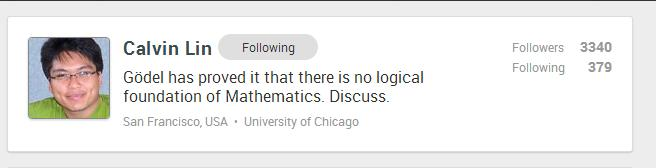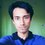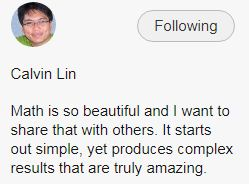# Gödel's Incomplete TheoremsThis is Calvin Lin's current status:

Gödel has proved it that there is no logical foundation of Mathematics. Discuss.

So, discuss!Note by Mursalin Habib
6 years, 1 month ago

This discussion board is a place to discuss our Daily Challenges and the math and science related to those challenges. Explanations are more than just a solution — they should explain the steps and thinking strategies that you used to obtain the solution. Comments should further the discussion of math and science.

When posting on Brilliant:

• Use the emojis to react to an explanation, whether you're congratulating a job well done , or just really confused .
• Ask specific questions about the challenge or the steps in somebody's explanation. Well-posed questions can add a lot to the discussion, but posting "I don't understand!" doesn't help anyone.
• Try to contribute something new to the discussion, whether it is an extension, generalization or other idea related to the challenge.

MarkdownAppears as
*italics* or _italics_ italics
**bold** or __bold__ bold
- bulleted- list
• bulleted
• list
1. numbered2. list
1. numbered
2. list
Note: you must add a full line of space before and after lists for them to show up correctly
paragraph 1paragraph 2

paragraph 1

paragraph 2

[example link](https://brilliant.org)example link
> This is a quote
This is a quote
    # I indented these lines
# 4 spaces, and now they show
# up as a code block.

print "hello world"
# I indented these lines
# 4 spaces, and now they show
# up as a code block.

print "hello world"
MathAppears as
Remember to wrap math in $$ ... $$ or $ ... $ to ensure proper formatting.
2 \times 3 $2 \times 3$
2^{34} $2^{34}$
a_{i-1} $a_{i-1}$
\frac{2}{3} $\frac{2}{3}$
\sqrt{2} $\sqrt{2}$
\sum_{i=1}^3 $\sum_{i=1}^3$
\sin \theta $\sin \theta$
\boxed{123} $\boxed{123}$

Sort by:

Now @Calvin Lin's current status:- 2 years, 6 months ago

Great status, nice...

- 4 years ago

Oh, this has just been reshared. Well, let me jump right in. Godel's Incompleness Theorems is a popular subject of discussion, but what's not mentioned often enough is the connection with Turing's Halting Problem. For people familiar with computer programming, it might be easier to understand the former in terms of the latter. Anyone who has done programming knows how easily it happens for a program that hasn't been debugged to "get stuck in some kind of an endless loop", forcing a reboot. It's not true that programs that crash do so because it's in an endless loop, but what can happen is that the program never halts, and we have no idea if it ever will. Turing was the first to prove that there exists no algorithm or systematic method of determining whether or not any given code would not suffer from this, thus condemning generations of programmers to the everlasting worry that their code may fail. Numerous programming techniques and restrictions on how code may be written and compiled have been advanced to "stay within the box of coding that are known not to suffer from this halting problem". The analogy here in mathematics is that mathematicians work to identify theorems that are proven, or problems that are known to have solutions (but yet found). But unfortunately, just as with programmers not having that confidence in arbitrarily written coding won't fail, mathematicians have to live with the reality that there are many conjectures, the proofs of which may "never halt", i.e., not determinable in a finite time.

However, to make the claim, "There are no logical foundation of Mathematics" is throwing the baby out with the bathwater. Just as modern programmers have to work with coding principles and restrictions to avoid the halting problems, mathematicians simply have to accept that there is a smaller subset of "all possible conjectures" that either have proofs or at least not shown to be undecidable, that make for the mathematics we have and can use, and relegate the rest to the Oort Cloud of undecidables. There's still a practical, useful mathematics, and there's a foundation for it, within that limited sphere. It had been an illusion to begin with that any utterable conjecture necessarily is either true or false (the Law of Excluded Middle---which had to be made an AXIOM), so now, as with the discovery of Non-Euclidean Geometry and its utility, we have a wider, and perhaps richer understanding of mathematics, one that affords more possibilities, not less.

- 5 years, 10 months ago

This makes me think of the Euclid's Elements, and his 5 axioms.

- 6 years, 1 month ago

Heheh.

- 6 years, 1 month ago

Whaaaa?

- 6 years, 1 month ago

I don't even want to think about that. $1+1=2$. Bam. Done.

- 6 years, 1 month ago

Although, you could "prove" that $1+1=2$... Hmm...

- 6 years, 1 month ago

There is a proof that 1=2, but it's rather faulty.

- 6 years, 1 month ago

Yeah...... I saw a mind blowing proof somewhere on this site!

- 6 years, 1 month ago

$\textit{Principia Mathematica}$ says "The above proposition is occasionally useful." when referring to that equation.

- 6 years, 1 month ago

Try changing bases. :-0 It won't stay consistent, I think that's kinda what it says. Perhaps...

- 6 years, 1 month ago

Not really, what it refers to is the fact that you must take certain things on faith, you can't prove it i.e. axioms. When changing bases arithmetic is still consistent, you can do any calculation in any base and still get the same answer, just in a different base. 1+1=10 in base 2, however 10 in base 2 equals 2 in base 10, you still get the same answer, but it just "looks" different because they are in different bases.

- 6 years, 1 month ago

That's... certainly correct.

- 6 years, 1 month ago

I've had this on a math test.

"What is $1+1?$ Base $10,$ no tricks!"

The answer is $10$ because the entire question was written in base $2,$ including the part that told you what the base was. I was really happy when I got that. (It was extra credit)

- 6 years, 1 month ago

That's awesome. :D

- 6 years, 1 month ago

I agree

- 6 years, 1 month ago

Ha, ha funny.

- 6 years, 1 month ago

Let me get the ball rolling

Godel's incompleteness theorem explains that there are inherent limitations in mathematical structure. In particular, it shows that there is no proof which demonstrates that arithmetic is consistent.

The first incompleteness theorem states that no consistent system of axioms whose theorems can be listed by an "effective procedure" (e.g., a computer program, but it could be any sort of algorithm) is capable of proving all truths about the relations of the natural numbers (arithmetic). For any such system, there will always be statements about the natural numbers that are true, but that are unprovable within the system.

Food for thought: If we were asked to prove a given statement, but are unable to do so after an extended period of time, is it possible that the statement is unprovable?

The second incompleteness theorem, an extension of the first, shows that such a system cannot demonstrate its own consistency. This tells us that there are statements, in which we do not know if such a statement could be proved within the system. A possible candidate for such a statement, is the Riemann Hypothesis. There have been proofs of a statement X, which first consider the case where the Riemann hypothesis is true and demonstrates X, and next consider the case where the Riemann hypothesis is false and demonstrates X.

Food for thought: If we were asked to prove a given statement, but are unable to do so after an extended period of time, is it possible that we do not know if the statement is unprovable?

Staff - 6 years, 1 month ago

@Calvin Lin I have some questions as well.

(1) Does a statement have to be either true or false?

What about statements like "This statement is false"?

(2) If we prove that a statement $A$ can not be false, is it okay to say that we've proved $A$ to be true?

We do that all the time, don't we? When we prove that $\sqrt{2}$ is irrational, we show that the assumption that $\sqrt{2}$ is a rational number leads to a contradiction and therefore $\sqrt{2}$ can not be rational.

Finally the most important question:

(3) What is wrong with the following reasoning?

From what I understand about the incompleteness theorems, the first theorem is proved by constructing a 'Gödel Sentence', $G$, which goes along the way of "This statement is unprovable given this set of axioms". Now this statement can't be false because that would cause a paradox. In other words, it is impossible for $G$ to be false. Now if the answer to question (2) is yes, then doesn't it mean that we've proved $G$ to be true? But that would lead to a paradox as well! So, $G$ is neither true nor false, very much like the statement in question (1).

So is there anything wrong with this reasoning? I should add that I don't have much background on the incompleteness theorems, so I could be wrong.

- 6 years, 1 month ago

I'd suppose, I'd agree with both of those questions. Either that or with the current level of our mathematics, we can't seem to prove something. Or perhaps we might require to change some definitions of things to prove them. Hmm...

- 6 years, 1 month ago